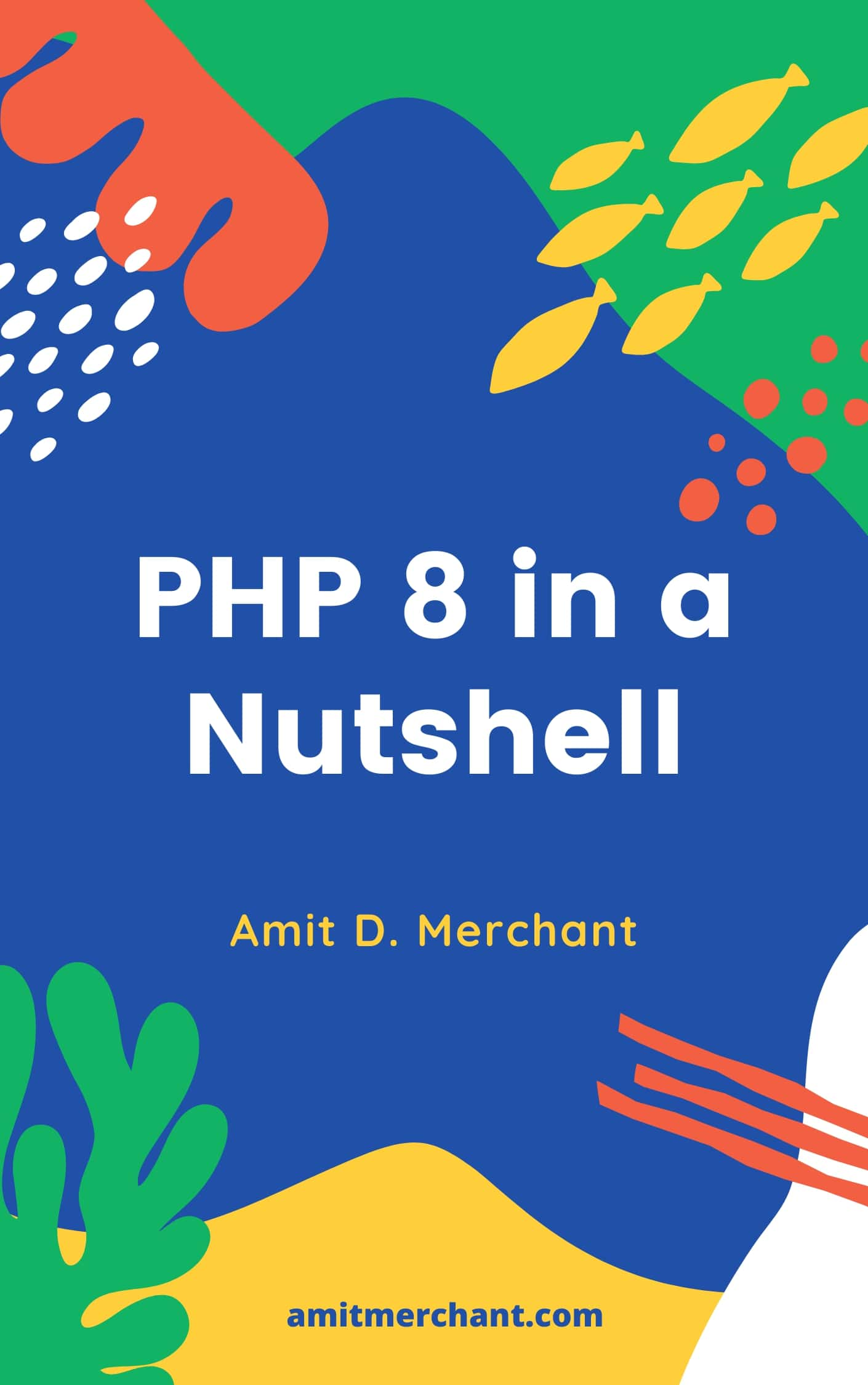# Amit MerchantVerified (\$10/year for the domain)

A blog on PHP, JavaScript, and more

# Rest vs Spread syntax in JavaScript

I recently wrote an article on how to conditionally spread objects in JavaScript. In that article, I mentioned that the spread operator (`...`) can be used to spread the properties of an object.

You can check out that article. But in this article, I’m going to talk about the rest operator (`...`) which looks similar to the spread operator but is used for a different purpose. We’ll see how these two operators are different from each other.

And since both these operators look the same, I have seen people call them out interchangeably. So, let’s decipher this confusion.

The spread operator (`...`) is used to spread the properties of an object. For example, you can do something like this.

``````const obj = {
a: 1,
b: 2,
c: 3
};

const newObj = {
...obj
};

console.log(newObj);
// { a: 1, b: 2, c: 3 }
``````

As you can see, the `newObj` object has all the properties of the `obj` object. This is because of the spread operator (`...`) which is used to spread the properties of the `obj` object.

As the name suggests, the spread operator “spreads” the properties of an object to another object. So, it’s pretty simple to remember.

One more use case of the spread operator is to spread the function arguments when calling the function. For example, you can do something like this.

``````const arr = [1, 2, 3];

const sum = (a, b, c) => a + b + c;

console.log(sum(...arr)); // Outputs: 6
//                 ^
//          sum(1, 2, 3)
``````

As you can see, the spread operator (`...`) is used to spread the array elements to the function arguments. So, the `sum()` function will receive the array elements as the function arguments.

## Rest operator

On the other hand,

The rest operator (`...`) is used to collect the function arguments into an array. So, it’s the opposite of the spread operator.

For example, you can do something like this.

``````const sum = (...args) => {
return args.reduce((acc, curr) => acc + curr, 0);
//      ^
//  [1, 2, 3]
};

console.log(sum(1, 2, 3));
// 6
``````

As you can see, the rest operator (`...`) is used to collect the function arguments into an array. So, the `sum()` function will receive the function arguments as an array.

You can also use the rest operator to collect the remaining arguments of a function. For example, you can do something like this.

``````const sum = (a, b, ...restOfArguments) => {
return a + b + restOfArguments.reduce((acc, curr) => acc + curr, 0);
//     ^   ^          ^
//     1   2      [3, 4, 5]
};

console.log(sum(1, 2, 3, 4, 5));
// 15
``````

As you can tell, the rest operator (`...`) is used to collect the remaining arguments of the `sum()` function into an array. So, the `sum()` function will receive the remaining arguments as an array (`restOfArguments`) that you can use to loop through.

### Caveats

There are a few caveats with the rest operator. You can only use it as the last argument of a function. For example, you can’t do something like this.

``````const sum = (a, b, ...restOfArguments, c) => {
return a + b + restOfArguments.reduce((acc, curr) => acc + curr, 0) + c;
};

console.log(sum(1, 2, 3, 4, 5, 6));
// SyntaxError: Rest parameter must be last formal parameter
``````

Also, you can’t use multiple rest operators in a function. For example, you can’t do something like this.

``````const sum = (a, b, ...restOfArguments, ...restOfArguments2) => {
return a + b + restOfArguments.reduce((acc, curr) => acc + curr, 0) + restOfArguments2.reduce((acc, curr) => acc + curr, 0);
};

console.log(sum(1, 2, 3, 4, 5, 6, 7, 8, 9));
// SyntaxError: Rest parameter must be last formal parameter
``````

## Conclusion

In this article, we saw how visually same the spread operator (`...`) and the rest operator (`...`) are different from each other. We also saw how to use them in JavaScript.

Prefer video format instead? Fret not!

👋 Hi there! I'm Amit. I write articles about all things web development. If you like what I write and want me to continue doing the same, I would like you buy me some coffees. I'd highly appreciate that. Cheers!(Includes PHP 8.1 and 8.2)

My Tiny YouTube Channel ➔